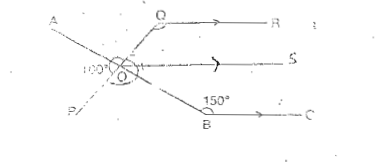# Primary 6 SA2 Practice #5

Time elapsed:
Q1. In the diagram below, the shaded square is ___ of the mosque     [1 mark]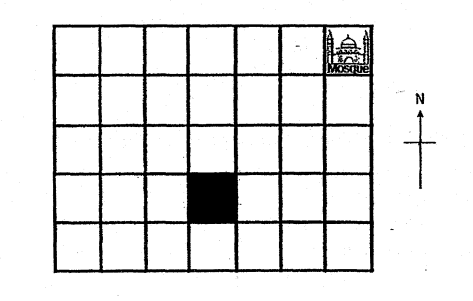Q2. An apple cost 35¢ and an orange cost 40¢ each. Ulysses bought 8 apples and 14 oranges. How many more apples can he buy if he were to spend all his money on apples?     [1 mark]
Q3. Which of the following is equal to 5 13?     [1 mark]
Q4. Grace and Paula had an average number of 140 stickers. After Jason joined in with some stickers, the average number of stickers became 154. How many stickers did Jason have?     [2 marks]
Q5. The table below shows the charges for bicycle rental. Shawn wants to rent a bicycle for 3 hours. How much does he need pay, including the deposit?     [2 marks]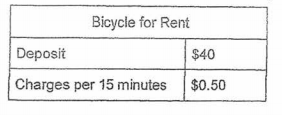Q6. How much water is in the container shown below?     [1 mark]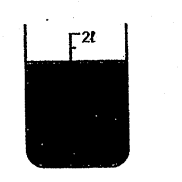Q7. 1 + 110 + 11000 = ___.     [1 mark]
Q8. Francis has the same number of twenty-cent coins and fifty-cent coins. Their total value is \$42. How many coins does Francis have altogether?     [1 mark]
Q9. During a promotion, Tasty Bakery gave away a free pancake for every 4 pancakes purchased. What was the percentage discount offered during the promotion?     [1 mark]
Q10. Which of the following is the same as 6.05 km?     [1 mark]
Q11. Round 123.456 to the nearest hundredth.     [1 mark]
Q12. Which of the following is the largest in value?     [1 mark]
Q13. Which of the following numbers is 12 000 when rounded to the nearest hundred?     [1 mark]
Q14. The opening hours of Chan's Clinic are shown below. How long is the clinic open each day?     [1 mark]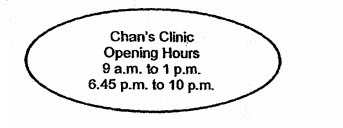Q15. Nathaniel weighs 3x kg. Alex is twice as heavy as Nathaniel. Kingston is 2x kg lighter than Alex. What is their total weight?     [2 marks]
Q16. A windmill turns 1500 rounds in a hour. How many rounds can it turn in 5 minutes?     [1 mark]
Q17. A piece of paper in the shape of an equilateral triangle is folded along dotted line as shown below. Find ∠x     [2 marks]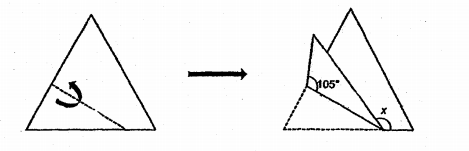Q18. In the scale below, what is the value of Y?     [1 mark]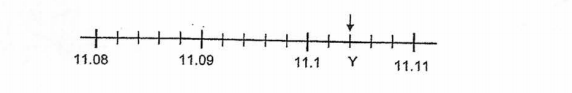Q19. Which of the following is the same as 9.04 ℓ?     [1 mark]
Q20. In the figure below, not drawn to scale, AOB and POQ are straight lines, QR // OS and OS // BC. Find ∠OQR.     [1 mark]